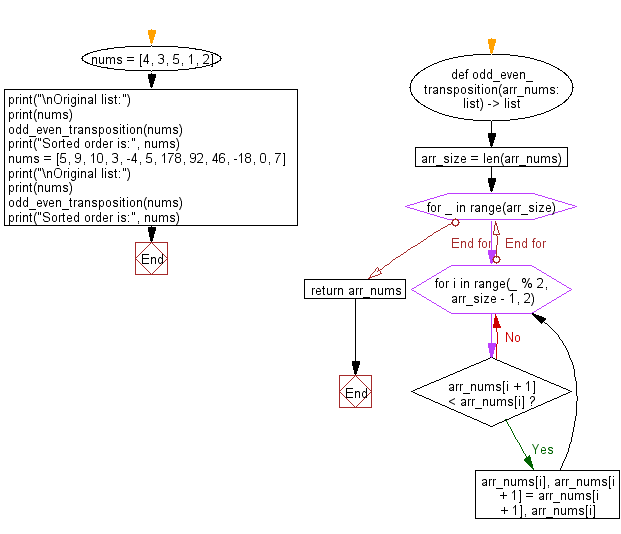﻿ Python: Sort unsorted numbers using non-parallelized implementation of odd-even transposition sort - w3resource# Python: Sort unsorted numbers using non-parallelized implementation of odd-even transposition sort

## Python Search and Sorting : Exercise-36 with Solution

Write a Python program to sort unsorted numbers using non-parallelized implementation of odd-even transposition sort.

From Wikipedia:
In computing, an odd-even sort or odd-even transposition sort (also known as brick sort or parity sort) is a relatively simple sorting algorithm, developed originally for use on parallel processors with local interconnections. It is a comparison sort related to bubble sort, with which it shares many characteristics. It functions by comparing all odd/even indexed pairs of adjacent elements in the list and, if a pair is in the wrong order (the first is larger than the second) the elements are switched. The next step repeats this for even/odd indexed pairs (of adjacent elements). Then it alternates between odd/even and even/odd steps until the list is sorted.

Sample Solution:

Python Code:

``````def odd_even_transposition(arr_nums: list) -> list:
arr_size = len(arr_nums)
for _ in range(arr_size):
for i in range(_ % 2, arr_size - 1, 2):
if arr_nums[i + 1] < arr_nums[i]:
arr_nums[i], arr_nums[i + 1] = arr_nums[i + 1], arr_nums[i]

return arr_nums

nums = [4, 3, 5, 1, 2]
print("\nOriginal list:")
print(nums)
odd_even_transposition(nums)
print("Sorted order is:", nums)
nums = [5, 9, 10, 3, -4, 5, 178, 92, 46, -18, 0, 7]
print("\nOriginal list:")
print(nums)
odd_even_transposition(nums)
print("Sorted order is:", nums)
```
```

Sample Output:

```Original list:
[4, 3, 5, 1, 2]
Sorted order is: [1, 2, 3, 4, 5]

Original list:
[5, 9, 10, 3, -4, 5, 178, 92, 46, -18, 0, 7]
Sorted order is: [-18, -4, 0, 3, 5, 5, 7, 9, 10, 46, 92, 178]
```

Flowchart:Python Code Editor:

What is the difficulty level of this exercise?

Test your Programming skills with w3resource's quiz.

﻿

## Python: Tips of the Day

The Zip() Function:

```>>> students = ('John', 'Mary', 'Mike')
>>> ages = (15, 17, 16)
>>> scores = (90, 88, 82, 17, 14)
>>> for student, age, score in zip(students, ages, scores):
...     print(f'{student}, age: {age}, score: {score}')
...
John, age: 15, score: 90
Mary, age: 17, score: 88
Mike, age: 16, score: 82
>>> zipped = zip(students, ages, scores)
>>> a, b, c = zip(*zipped)
>>> print(b)
(15, 17, 16)
```